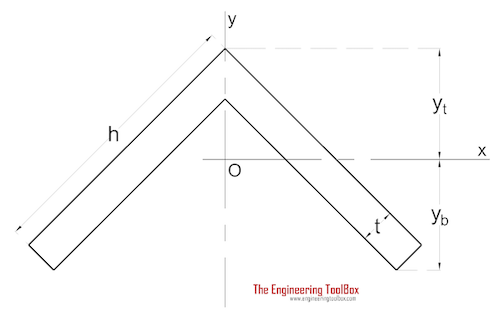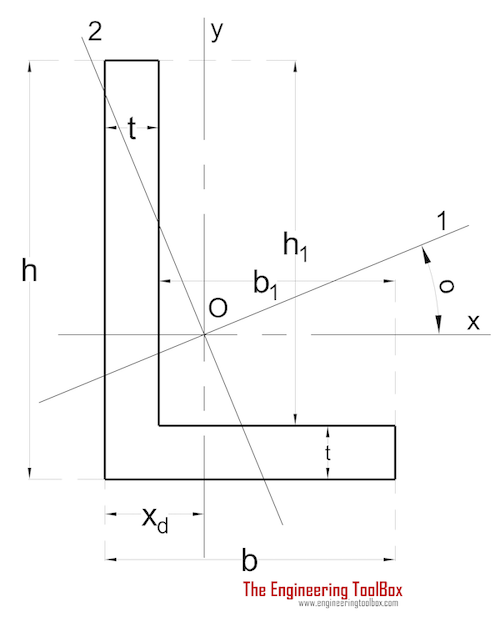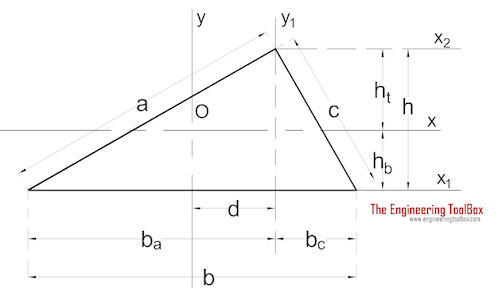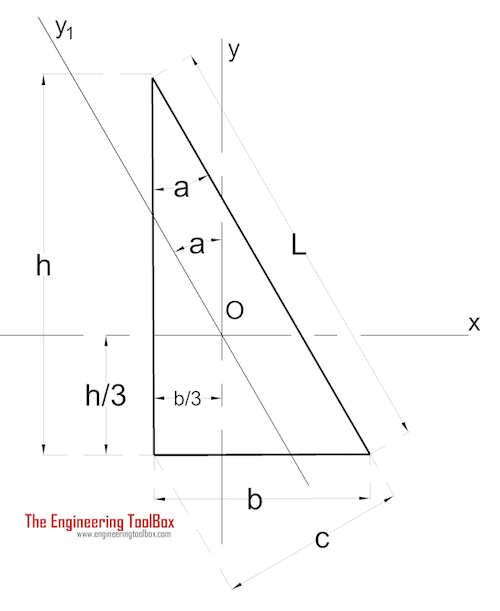Engineering ToolBox - Resources, Tools and Basic Information for Engineering and Design of Technical Applications!

# Area Moment of Inertia - Typical Cross Sections II

## Area Moment of Inertia, Moment of Inertia for an Area or Second Moment of Area for typical cross section profiles.

Area Moment of Inertia or Moment of Inertia for an Area - also known as Second Moment of Area - I, is a property of shape that is used to predict deflection, bending and stress in beams.

### Area Moment of Inertia for typical Cross Sections II

#### Angle with Equal LegsThe Area Moment of Inertia for an angle with equal legs can be calculated as

Ix = 1/3 [2c4 - 2 (c - t)4 + t (h - 2 c + 1/2 t)3]                       (1a)

where

c = yt cos 45o                             (1b)

and

yt = (h2 + ht + t2) / [2 (2 h - t ) cos 45o]                               (1c)

#### Angle with Unequal LegsThe Area Moment of Inertia for an angle with unequal legs can be calculated as

Ix = 1/3 [t (h - yd)3 + b yd3 - b1 (yd - t)3]                            (2a)

Iy = 1/3 [t (b - xd)3 + h xd3 - h1 (xd - t)3]                            (2b)

where

xd = (b2 + h1 t) / (2 (b + h1))                                 (2c)

yd = (h2 + b1 t) / (2 (h + b1))

#### TriangleThe Area Moment of Inertia for a triangle can be calculated as

Ix =  b h3 / 36                                (3a)

Iy = h b (b2 - ba bc) / 36                         (3b)

#### Rectangular TriangleThe Area Moment of Inertia for a rectangular triangle can be calculated as

Ix =  b h3 / 36                                (4a)

Iy =h b3 / 36                              (4b)

## Related Topics

• Beams and Columns - Deflection and stress, moment of inertia, section modulus and technical information of beams and columns.
• Mechanics - Forces, acceleration, displacement, vectors, motion, momentum, energy of objects and more.

## Engineering ToolBox - SketchUp Extension - Online 3D modeling!

Add standard and customized parametric components - like flange beams, lumbers, piping, stairs and more - to your Sketchup model with the Engineering ToolBox - SketchUp Extension - enabled for use with the amazing, fun and free SketchUp Make and SketchUp Pro .Add the Engineering ToolBox extension to your SketchUp from the SketchUp Pro Sketchup Extension Warehouse!

Translate

## Privacy

We don't collect information from our users. Only emails and answers are saved in our archive. Cookies are only used in the browser to improve user experience.

Some of our calculators and applications let you save application data to your local computer. These applications will - due to browser restrictions - send data between your browser and our server. We don't save this data.

## Citation

• Engineering ToolBox, (2003). Area Moment of Inertia - Typical Cross Sections II. [online] Available at: https://www.engineeringtoolbox.com/area-moment-inertia-d_521.html [Accessed Day Mo. Year].

Modify access date.

. .

#### Scientific Online Calculator6 24